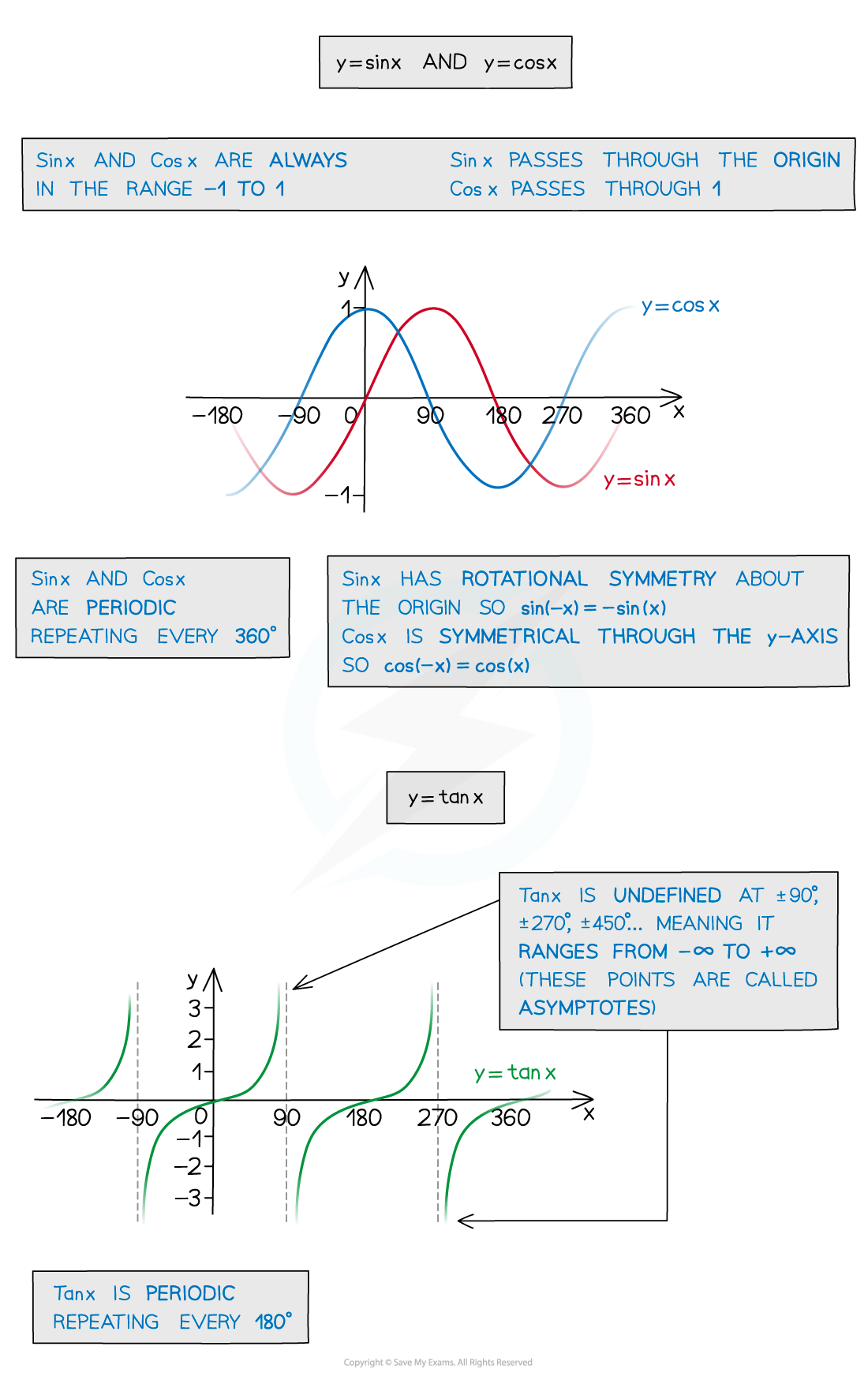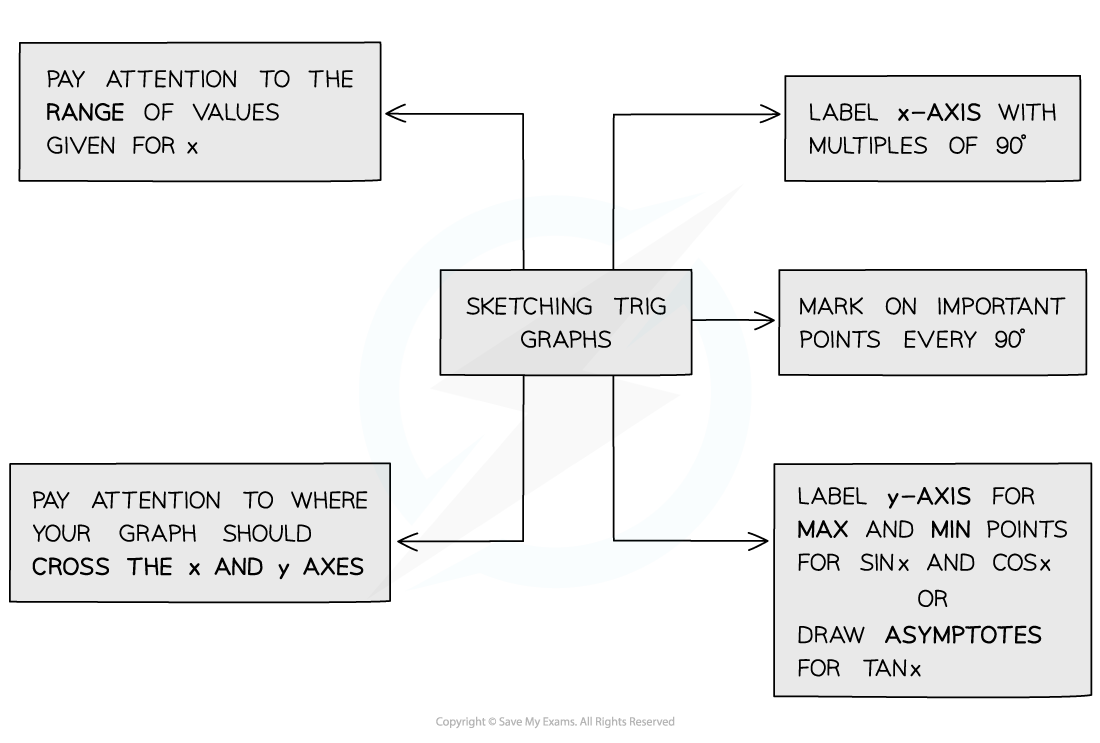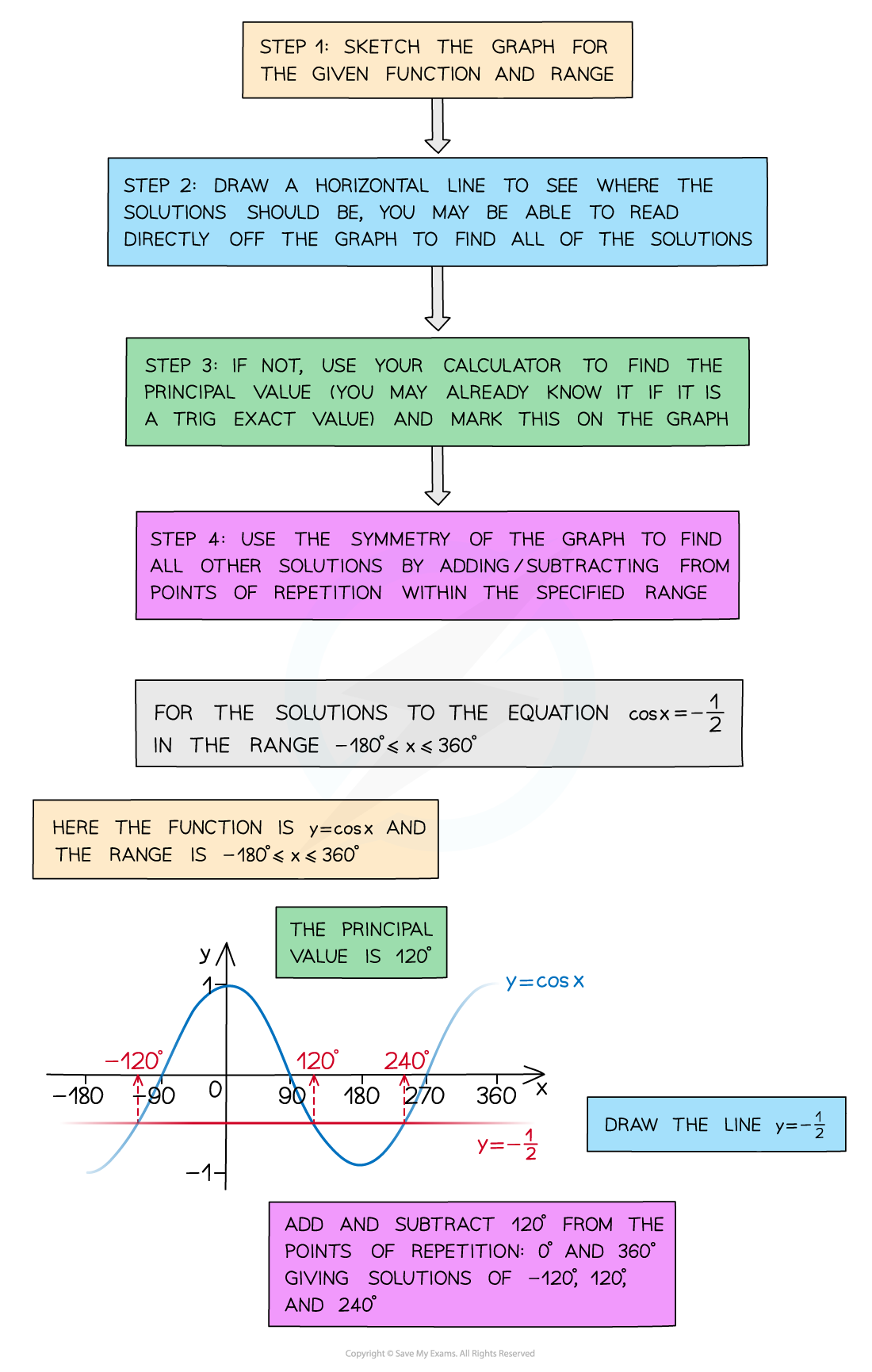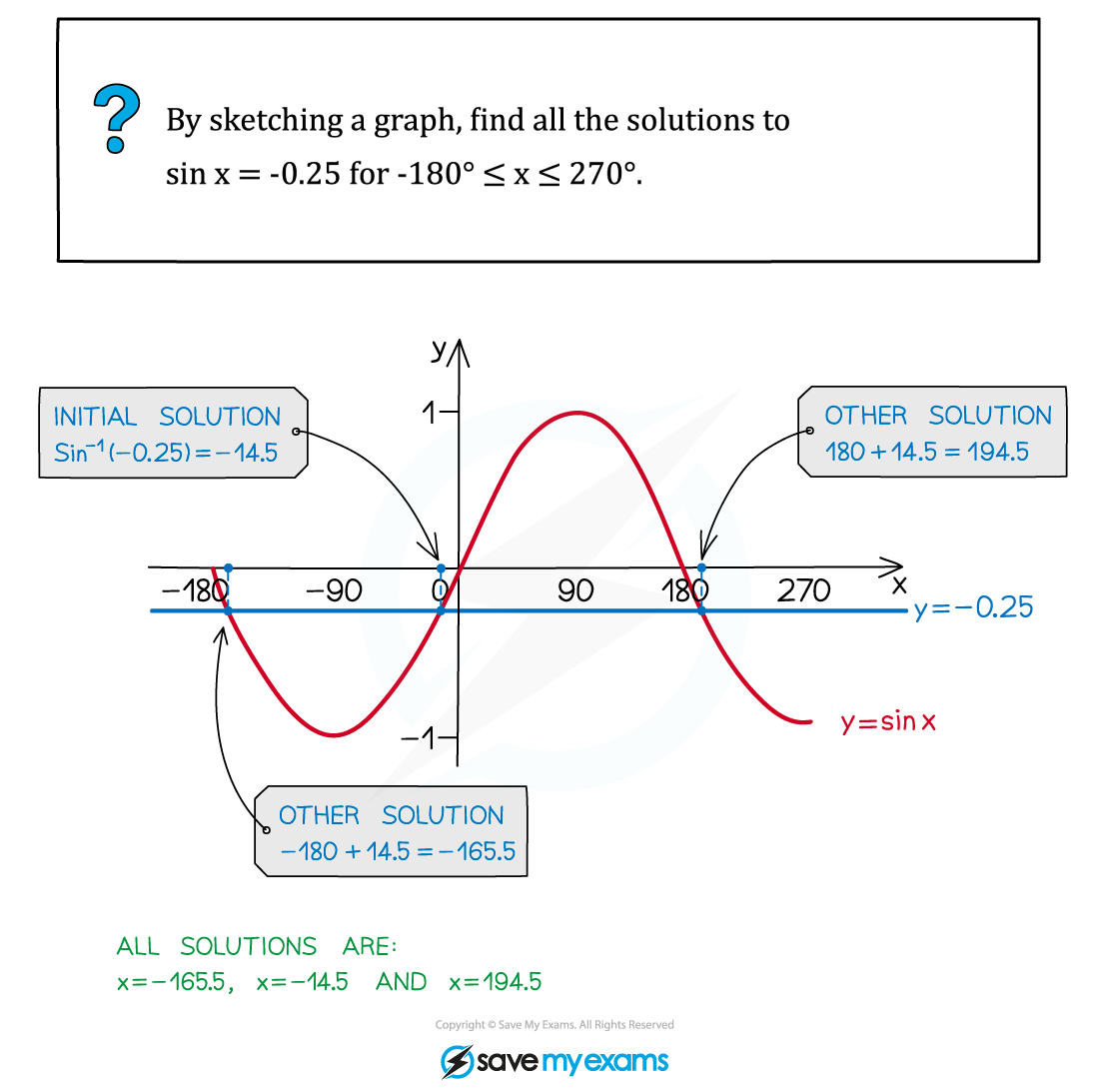# AQA A Level Maths: Pure复习笔记5.2.1 Graphs of Trigonometric Functions

### Graphs of Trigonometric Functions

#### Graphs of trigonometric functions

• The Trigonometric Functions Sin, Cos and Tan all have special periodic graphs that you need to be able to sketch and remember
• You’ll need to know their properties and how to sketch them to solve equations and for transforming trig functions#### Sketching trigonometric graphs#### Using trigonometric graphs

• By sketching the graph you can read off all the solutions in a given range (or interval)
• Your calculator will only give you the principal value
• You should recognise any values/angles associated with exact values
• You should be able to spot the pattern of solutions using the symmetry and periodicity of the graph#### Exam Tip

• Always sketch with a pencil and draw a smooth curve and pay attention to the key features of each graph:
• Where it crosses the x and y axes
• How often it repeats
• Whether it is symmetrical
• Remember, when answering exam questions that ask for solutions, a sketch will help ensure you give all the appropriate solutions for a given interval.

#### Worked Example